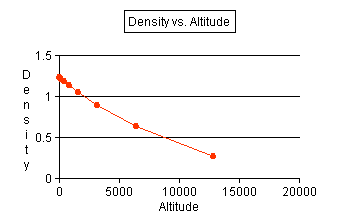+ Text Only Site
+ Non-Flash Version
+ Contact Glenn## Density ActivityAltitude (meters) Density (kg/m3) 0 1.22859 100 1.21686 200 1.20521 400 1.18217 800 1.13711 1600 1.05093 3200 0.89362 6400 0.63345 12800 0.27386

1. Use the data that you recorded to produce a line graph. Use altitude as the independent variable and make a line of best fit. See graph.2. Use your graph to make a general statement on the relationship of density to altitude. As altitude increases, the density decreases with a decreasing rate.
3. Why do you think this happens? The atoms and molecules in air are held close to the earth by gravity since it has a greater effect close to the surface. The farther away from the surface, the fewer particles there are; so the density is lower.
4. Convert the following altitudes from meters to feet.
a) 1000 meters = 3281 feet
b) 2500 meters = 8202 feet
c) 6500 meters = 21325 feet
5. After converting your altitudes to feet, you can calculate (or predict) the temperature at these altitudes by using the following equation: T = 59 - .00356 (h), where h = altitude (in feet). Your temperatures will be in degrees F. Calculate the temperature for each of the altitudes in Question 4.
a) T1 = 59 - .00356(3281) = 47.3*F
b) T2 = 59 - .00356(8202) = 29.8*F
c) T3 = 59 - .00356(21325) = -16.9*F
6. Use AtmosModeler to check your answers. Be sure the "Units" menu next says "Imperial" to compute in English units. Input each of the altitudes (in feet). Compare your answers for temperature to the AtmosModeler temperatures and explain how any differences may have occurred.
a) T1 = 47o F
b) T2 = 29o F
c) T3 = -17o F
T1 and T3 appear to be rounded correctly. But T2 is rounded down instead of up.
7. The temperature may now be used to calculate the pressure of the air at each altitude. Using the equation p = 2116 (T + 459.7 / 518.6)5.256 where T = degrees F and p = lbs/in2, find the pressure that corresponds to each of your temperatures.
a) T1 = 13.04 lb/sq in (89.86 K - Pascals)
b) T2 = 10.84 lb/sq in (74.7 K - Pascals)
c) T3 =6.4 lb/sq in (44.08 K - Pascals)
8. How does the pressure change compared to a change in altitude?
The pressure goes down as the altitude increases.
9. Use a model or table to compare the change in altitude, density, temperature, and pressure at the same time. Include an explanation.
 Altitude (feet) Density (slugs/ft3) Temperature (oF) Pressure (lbs/sq in) 3281 .00216 47 13.04 8202 .00186 29 10.84 21,325 .00121 -17 6.4 Increase Decrease Decrease Decrease
As the altitude increases, the density of the air decreases. This, in turn, causes the pressure to drop, as does the temperature.

Related Pages:
Standards
Activity
Worksheet
Lesson Index
Aerodynamics Index+ Inspector General Hotline + Equal Employment Opportunity Data Posted Pursuant to the No Fear Act + Budgets, Strategic Plans and Accountability Reports + Freedom of Information Act + The President's Management Agenda + NASA Privacy Statement, Disclaimer, and Accessibility CertificationEditor: Tom Benson NASA Official: Tom Benson Last Updated: Thu, Jun 12 04:47:14 PM EDT 2014 + Contact Glenn